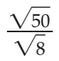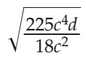### Day 07 - Exponential Growth/Decay - 08.26.14

 Bell RingerSimplify the following:square root of 6.255 / 2(square root of 5) / 2(square root of 5) / (square of 2)none of the aboveSimplify the following:See board for answer choices.See board for answer choices.See board for answer choices.See board for answer choices.none of the aboveWhich answer represents the exponential growth equation for the following scenario: In 1950, the use of coal was 114.6 million tons. Because businesses started using more and more cleaner source of energy, the use of coal has decreased by 6.6% each year.y = 114,600,000(1-0.066)ty = 1146(1-0.066)tt = 114,600,000(1-0.066)yy = 114.6(1-0.66)tnone of the aboveUsing the scenario from problem 3, what would we predict the amount of coal that will be used in 2015.1.35 million tons1 million tons1.35 tons14.6 million tonsReviewExponent PropertiesProduct of PowersPower of a PowerPower of a ProductQuotient of PowersPower of a QuotientZero ExponentNegative ExponentsRadical PropertiesProduct of Square RootsQuotient of Square RootsRationalizing the DenominatorRational ExponentsLessonExponential Growth and Decay Practice Problemspage 563 #1, 3, 7, 9, 10, 11-13, 18Exit TicketA car sells for \$16,000. If the rate of depreciation is 18% each year, what is the theoretical value of the car in 8 years?\$3270.63\$0.018\$60,141.75\$78,272.49In 2011, there were 185 rabbits in Central Park.  The population has increased by about 12% each year.  About how many rabbits were in Central Park in 2014?260 rabbits126 rabbits0.32 rabbits186 rabbitsA sample of bacteria double every 3 hours. Choose the equation that best fits the model for the growth of bacteria.y = 2t/3y = 2ty = 2ty = t2 Lesson Objective(s) How does linear growth/decay differ from exponential growth/decay? How can exponential growth/decay be modeled using mathematics? Standard(s) N.RN.1  Explain how the definition of the meaning of rational exponents follows from extending the properties of integer exponents to those values, allowing for a notation for radicals in terms of rational exponents. N.RN.2  Rewrite expressions involving radicals and rational exponents using the properties of exponents. A.CED.1 Create equations and inequalities in one variable and use them to solve problems.Include equations arising from linear and quadratic functions, and exponential functions. Mathematical Practice(s) #2: Reason abstractly and quantitatively Students will use concrete examples of numerical manipulation to examine closure of rational and irrational numbers. For example, students will use numeric examples of sums and products of rational numbers to generalize the closure of rational numbers under addition and multiplication. #4: Model with mathematics Students will create equations using rational or radical expressions to represent mathematical models of real-world situations like interest rates or depreciation. Summary of Concepts